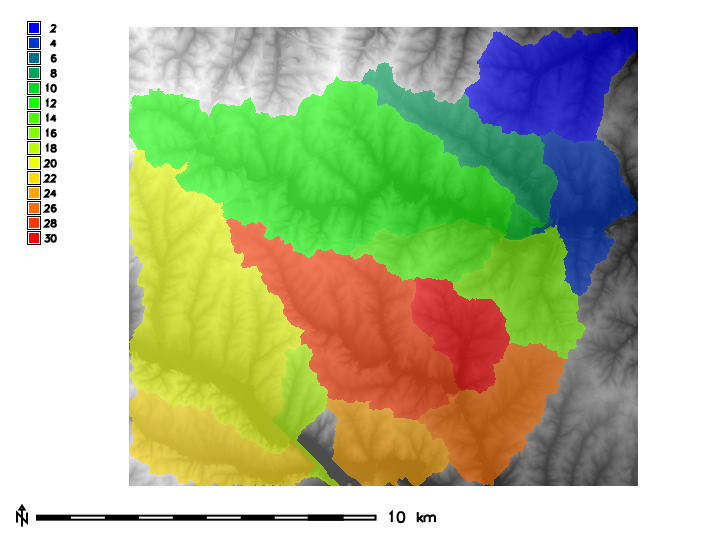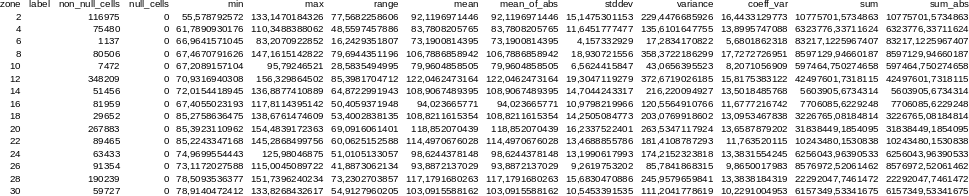Note: A new GRASS GIS stable version has been released: GRASS GIS 7.6, available here.
Updated manual page: here

## NAME

r.univar - Calculates univariate statistics from the non-null cells of a raster map.
Statistics include number of cells counted, minimum and maximum cell values, range, arithmetic mean, population variance, standard deviation, coefficient of variation, and sum.

## KEYWORDS

raster, statistics, univariate statistics, zonal statistics

## SYNOPSIS

r.univar
r.univar --help
r.univar [-getr] map=name[,name,...] [zones=name] [output=name] [percentile=float[,float,...]] [separator=character] [--overwrite] [--help] [--verbose] [--quiet] [--ui]

### Flags:

-g
Print the stats in shell script style
-e
Calculate extended statistics
-t
Table output format instead of standard output format
-r
Use the native resolution and extent of the raster map, instead of the current region
--overwrite
Allow output files to overwrite existing files
--help
Print usage summary
--verbose
Verbose module output
--quiet
Quiet module output
--ui
Force launching GUI dialog

### Parameters:

map=name[,name,...] [required]
Name of raster map(s)
zones=name
Raster map used for zoning, must be of type CELL
output=name
Name for output file (if omitted or "-" output to stdout)
percentile=float[,float,...]
Percentile to calculate (requires extended statistics flag)
Options: 0-100
Default: 90
separator=character
Field separator
Special characters: pipe, comma, space, tab, newline
Default: pipe

## DESCRIPTION

r.univar calculates the univariate statistics of one or several raster map(s). This includes the number of cells counted, minimum and maximum cell values, range, arithmetic mean, population variance, standard deviation, coefficient of variation, and sum. Statistics are calculated separately for every category/zone found in the zones input map if given. If the -e extended statistics flag is given the 1st quartile, median, 3rd quartile, and given percentile are calculated. If the -g flag is given the results are presented in a format suitable for use in a shell script. If the -t flag is given the results are presented in tabular format with the given field separator. The table can immediately be converted to a vector attribute table which can then be linked to a vector, e.g. the vector that was rasterized to create the zones input raster.

When multiple input maps are given to r.univar, the overall statistics are calculated. This is useful for a time series of the same variable, as well as for the case of a segmented/tiled dataset. Allowing multiple raster maps to be specified saves the user from using a temporary raster map for the result of r.series or r.patch.

## NOTES

As with most GRASS raster modules, r.univar operates on the raster array defined by the current region settings, not the original extent and resolution of the input map. See g.region, but also the wiki page on the computational region to understand the impact of the region settings on the calculations.

This module can use large amounts of system memory when the -e extended statistics flag is used with a very large region setting. If the region is too large the module should exit gracefully with a memory allocation error. Basic statistics can be calculated using any size input region. Extended statistics can be calculated using r.stats.quantile.

Without a zones input raster, the r.quantile module will be significantly more efficient for calculating percentiles with large maps.

For calculating univariate statistics from a raster map based on vector polygon map and uploads statistics to new attribute columns, see v.rast.stats.

## EXAMPLES

### Univariate statistics

In this example, the raster map elevation in the North Carolina sample dataset is used to calculate univariate statistics:
```g.region raster=elevation -p

# standard output, along with extended statistics
r.univar -e elevation percentile=98
total null and non-null cells: 2025000
total null cells: 0

Of the non-null cells:
----------------------
n: 2025000
minimum: 55.5788
maximum: 156.33
range: 100.751
mean: 110.375
mean of absolute values: 110.375
standard deviation: 20.3153
variance: 412.712
variation coefficient: 18.4057 %
sum: 223510266.558102
1st quartile: 94.79
median (even number of cells): 108.88
3rd quartile: 126.792
98th percentile: 147.727

# script style output, along with extended statistics
r.univar -ge elevation percentile=98
n=2025000
null_cells=0
cells=2025000
min=55.5787925720215
max=156.329864501953
range=100.751071929932
mean=110.375440275606
mean_of_abs=110.375440275606
stddev=20.3153233205981
variance=412.712361620436
coeff_var=18.4056555243368
sum=223510266.558102
first_quartile=94.79
median=108.88
third_quartile=126.792
percentile_98=147.727
```

### Zonal statistics

In this example, the raster polygon map basins in the North Carolina sample dataset is used to calculate raster statistics for zones for elevation raster map:
```g.region raster=basins -p
```
This will set and print computational region in the format:
```projection: 99 (Lambert Conformal Conic)
zone:       0
ellipsoid:  a=6378137 es=0.006694380022900787
north:      228500
south:      215000
west:       630000
east:       645000
nsres:      10
ewres:      10
rows:       1350
cols:       1500
cells:      2025000
```
Check basin's IDs using:
```r.category basins
```
This will print them in the format:
```2
4
6
8
10
12
14
16
18
20
22
24
26
28
30
```
Visualization of them underlying elevation map can be created as:
```d.mon wx0
d.rast map=elevation
r.colors map=elevation color=grey
d.rast map=basins
r.colors map=basins color=bgyr
d.legend raster=basins use=2,4,6,8,10,12,14,16,18,20,22,24,26,28,30
d.barscale
```Figure: Zones (basins, opacity: 60%) with underlying elevation map for North Carolina sample dataset.

Then statistics for elevation can be calculated separately for every zone, i.e. basin found in the zones parameter:

```r.univar -t map=elevation zones=basins separator=comma \
output=basin_elev_zonal.csv
```
This will print information in the format:
```zone,label,non_null_cells,null_cells,min,max,range,mean,mean_of_abs,
stddev,variance,coeff_var,sum,sum_abs2,,116975,0,55.5787925720215,
133.147018432617,77.5682258605957,92.1196971445722,92.1196971445722,
15.1475301152556,229.447668592576,16.4433129773355,10775701.5734863,
10775701.57348634,,75480,0,61.7890930175781,110.348838806152,
48.5597457885742,83.7808205765268,83.7808205765268,11.6451777476995,
135.610164775515,13.8995747088232,6323776.33711624,6323776.33711624
6,,1137,0,66.9641571044922,83.2070922851562,16.2429351806641,
73.1900814395257,73.1900814395257,4.15733292896409,17.2834170822492,
5.68018623179036,83217.1225967407,83217.12259674078,,80506,
0,67.4670791625977,147.161514282227, ...
```
Comma Separated Values (CSV) file is best viewed through a spreadsheet program such as Microsoft Excel, Libre/Open Office Calc or Google Docs:Figure: Raster statistics for zones (basins, North Carolina sample dataset) viewed through Libre/Open Office Calc.

## TODO

To be implemented mode, skewness, kurtosis.

g.region, r3.univar, r.mode, r.quantile, r.series, r.stats, r.stats.quantile, r.stats.zonal, r.statistics, v.rast.stats, v.univar

## AUTHORS

Hamish Bowman, Otago University, New Zealand
Extended statistics by Martin Landa
Multiple input map support by Ivan Shmakov
Zonal loop by Markus Metz

Last changed: \$Date\$

## SOURCE CODE

Available at: r.univar source code (history)

Note: A new GRASS GIS stable version has been released: GRASS GIS 7.6, available here.
Updated manual page: here

© 2003-2019 GRASS Development Team, GRASS GIS 7.4.5dev Reference Manual